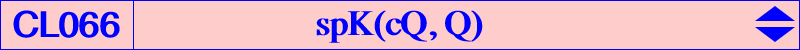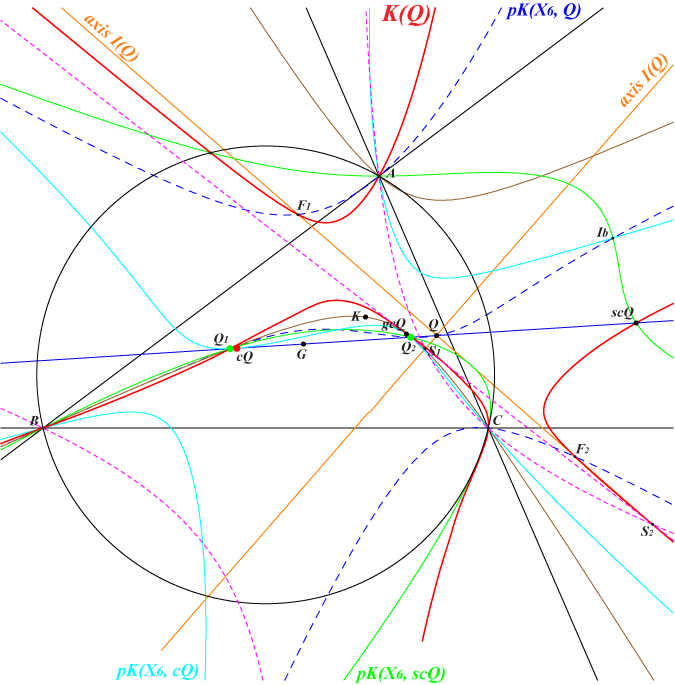Let Q be a point and K(Q) = spK(cQ, Q), where cQ is the complement of Q. See CL055 for general properties of spK cubics. When Q is the centroid G of ABC, K(Q) = spK(G, G) = pK(X6, G) = K002. This case is excluded in the sequel. When Q lies on the line at infinity, here again cQ = Q and K(Q) is a circular cubic. See below. For any point Q, K(Q) is a circum-cubic that must pass through : • four fixed points R0, R1, R2, R3 (independent of Q) described in the page K755. These are the common points of all the polar conics of the circumcenter O of ABC in the isogonal circular pKs. See CL035. Since these polar conics are rectangular hyperbolas, R0, R1, R2, R3 are the vertices of an orthocentric quadrilateral with diagonal triangle T whose circumcircle is C(X4, 2R) and whose in/excenters are these four points. Further details are given at K755. • the isogonal conjugate gcQ of cQ. • the reflection scQ of cQ in Q. • the infinite points of pK(X6, cQ). • the points on (O) of pK(X6, scQ). • the four foci of the inconic I(Q) with center Q (when Q is not an in/excenter nor a point at infinity). These foci lie on pK(X6, Q). The real foci are denoted F1, F2. • the two remaining common points S1, S2 of the axes of I(Q). These lie on a line passing through gcQ. • the traces on the sidelines of ABC of the reflections (Da), (Db), (Dc) in Q of the corresponding cevian lines of cQ. This gives a form of the equation of K(Q) which is ∑ a^2 y z (Da). • the common points Q1, Q2 of the line GQ and the conic which is its isogonal transform. Obviously, Q1, Q2 lie on K002.Special cubics spK(cQ, Q) • Circular cubics : these are the cubics obtained when Q lies on the line at infinity. K(Q) passes through Q, gQ. The singular focus is the homothetic of gQ under h(O, 5) hence it lies on the circle C(O, 5R). • Equilateral cubics : there is only one such cubic since cQ must be O hence Q = H. The cubic is the stelloid K525. • K0s : the term in xyz vanishes if and ony if Q lies on the line GK in which case K(Q) passes through G and K. See yellow cells in the table below and specially K755. • psKs : Q must lie on one median of ABC. The only pK is K(G) = K002. • nKs : cQ must lie on a nK(X2, X2, ?) passing through no listed ETC centers. • nodal cubics : Q must lie on a very complicated 12th degree curve passing through the in/excenters. • K+ : K(Q) has concurring asymptotes if and only if Q lies on K917, a circum-cubic with node H. K(H) is K525 as already mentioned. K(A), K(B), K(C) are central psK cubics with centers A, B, C respectively. • K(Q) passes through cQ when Q lies on K007. • When cQ lies on K007, K(Q) meets the sidelines of ABC again at the vertices of a pedal triangle. Some examples are given in the table below.The following table gives a selection of cubics K(Q), with contributions by Peter Moses.Q = X(i) X(i) on the cubic for i = Other remarkable points cubic / remark 1 1,58,79,3244,5563,10481 K915 2 see page midpoints, Thomson triangle K002 3 3,4,54,550 infinite points of K005 4 3,4,382 K525, central stelloid 5 3,4,546,1173 foci MacBeath inconic 6 2,6,61,62,251,3629,9484 foci orthic inconic K755 20 3,4,3529,12163 pedal triangle of X(3529), infinite points of K006 30 3,4,30,74 circular cubic 141 2,6,3108,3631 144 8,9,55,57,3062,7991 pedal triangle of X(7991) 149 79,513,3307,3308 193 2,6,25,3146,11008 pedal triangle of X(3146), infinite points of K169 K916 323 2,6,30,10594 385 2,6,1976,5984 395 2,6,14,16 396 2,6,13,15 524 2,6,111,524,8596 circular cubic 527 9,57,527,2291 circular cubic 631 3,4 CircumNormal triangle 1654 2,6,8,42 2475 3,4,8,65,79,5903 2895 2,6,37,5904 3218 9,57,80,84,909,1797 3219 9,40,57,1839,2259,3746 3448 523,1173,2574,2575 3522 3,4,20 antipodal triangle of ABC K918, central cubic 3616 1 circumcevian triangle of X(1) 3618 2,6 Grebe triangle 3832 3,4,3339,7982 5942 33,223,282,5691 7585 2,6,371,6420 7586 2,6,372,6419 7779 2,6,511,694,7772 8972 2,6,485,12818 10025 9,57,516,2195 13941 2,6,486,12819Remark : the cubics K(Q) are the members of a same net which contains several remarkable pencils corresponding to Q lying on a same line passing through G. Each pencil contains K002 and a circular pK. They are denoted by cells of the same color in the table.